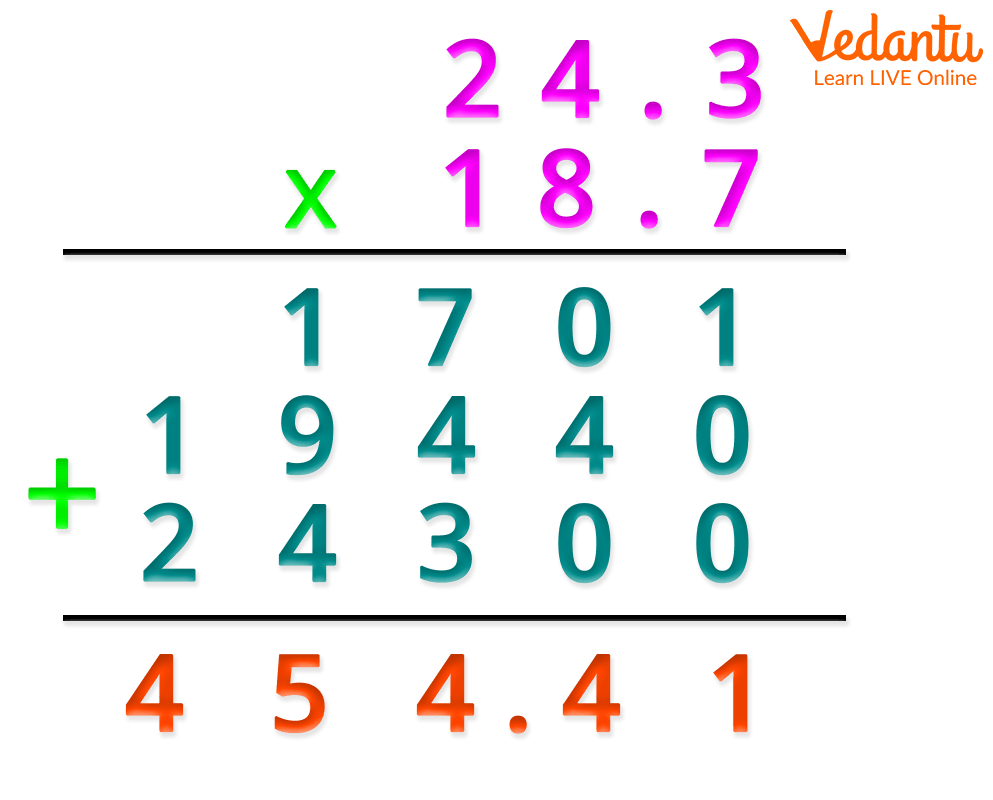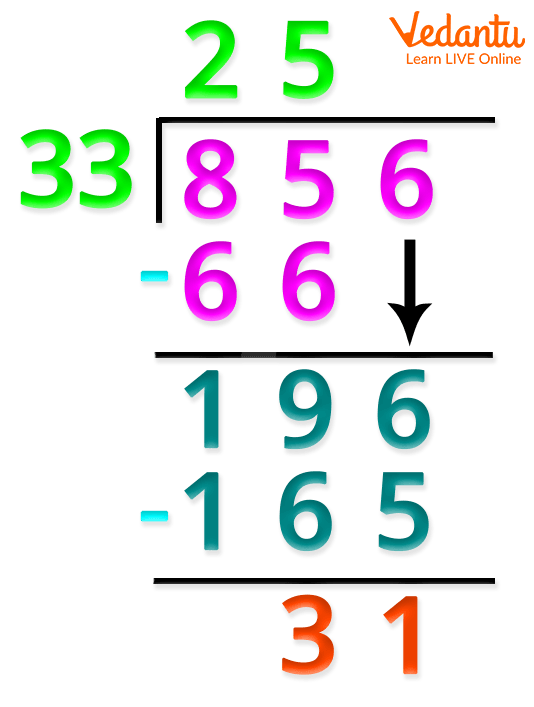Courses
Courses for Kids
Free study material
Free LIVE classes
More

# Compatible NumbersLIVE
Join Vedantu’s FREE Mastercalss

## Compatible Numbers Definition

Compatible numbers are defined as the numbers that are easy or compatible. It means the numbers that are easy to add, subtract, multiply, or divide are compatible. Also, these numbers are close in value to the actual numbers that make estimating the answer and solving problems easier.

For instance, if we add 485 and 549, we get our answer as 1034. Now, replace the number 549 by 550 and 485 to 480. Now, we add 550 and 480, we get our answer as 1030.

Here, our new answer 1030 is close to the actual number, i.e., 1034. This is how the concept of compatible numbers works.

Now, we must remember that these numbers are not only whole numbers but also there are decimal numbers too.

Here, on this page, you will get to know all the facts along with solved examples on the concept of compatible numbers.

## Compatible Numbers with Decimals

Let us take two numbers 639.3 and 376.2 and find the difference between these two.

639.3 – 376.2 = 263.1

The value 263.1 is the actual difference. Now, let us find the compatible difference:

After rounding off 639.3 and 376.2, we get 640 and 380.

So, the compatible difference is 640 – 380 = 260.

Here, we find that 260 is close to the actual value, i.e., 263.1.

## Compatible Fractions

Now, we will estimate fractions using compatible numbers by multiplying them.

For example, $\frac{3}{9}×11$.

Now, we will round off 11 to 12.

=>$\frac{3}{9}×12$

=> 4

Another problem we have is $\frac{5}{16}×20$, and solve it in the following way:

$\frac{5}{16}$ can be made compatible with $\frac{4}{16} = \frac{1}{4}$.

Now, $\frac{1}{4}$x 20 = 5 is the compatible fraction.

## Compatible Numbers in Multiplication

Assume that Car A travels at a speed of 24.3 km/hr and Car B with 18.7, then what is the product of these two speeds? Well, let us see the image solution of these two numbers:Compatible Numbers in Multiplication

Here, we get the actual multiplied result, and to determine the compatible multiplication value, we round-off 24.3 to 24 and 18.7 to 19, which is as follows:

24 x (10 + 9)

24 x 10 + 24 x 9

Now, 24 x 10 = 240, and

24 x 9 = 216.

Now, adding 240 and 216, we get 456. So, the compatible multiplied value is 456.

Here, the value 456 is close to the actual value 454.41; this is how compatible numbers in math works.

Now, let us do division using compatible numbers.

## Use Compatible Numbers to Estimate the Whole Number Quotient

Divide the whole number 856 by another whole number 33.

Below is the image solution:Compatible Numbers to Estimate the Whole Number Quotient

So, the whole number quotient for this problem is 25, which is the actual value.

Now, let us make compatible numbers from 856 to 900 and 33 to 30 and divide 900 by 30, we get 30 as our answer.

Here, 30 is close to the actual value 31. This is how compatible numbers for division work.

Hence, this was all about using compatible numbers to determine the addition, subtraction, multiplication, and division to find the close to value of the actual value.

Last updated date: 24th Sep 2023
Total views: 127.2k
Views today: 2.27k

## FAQs on Compatible Numbers

1. Why do we say compatible numbers are friendly numbers?

Compatible numbers are "friendly numbers'' because they make our calculations easier. Fifth grade students can find this topic easier in estimating the answer to questions.

For example, we have a number 2012 divided by 98 to get the quotient. Here, we round off 2012 to 2,000 and 98 to 100. Further, divide 2,000 by 100, we get 20 as our answer.

2. How can you explain compatible numbers to children?

Well, it is easy for them to make numbers as their friends. Assume that you mark an end-point 2km away from the pipe. Switch on the tap and water starts flowing. Now, what happens here is water crosses the end-point and reaches around 2.2 km.

Now, another pipe reaches 3.5 km. In both cases, we round-off 2.2 to 2 and 3.5 to 3.

Adding the two values together, we get a compatible sum as 5 km.